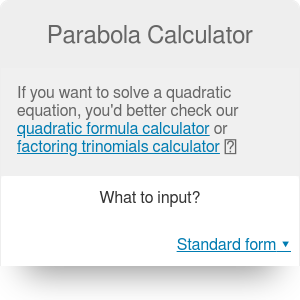Standard form: y = ax² + bx + c
a
b
c
Vertex form: y = a(x-h)² + k
h
k
Parabola focus
x-coordinate
y-coordinate
Parabola directrix
Directrix

# Parabola Calculator

By Bogna Haponiuk

Any time you come across a quadratic formula you want to analyze, this parabola calculator will be the perfect tool for you. Not only will it provide you with the parabola equation in the standard form and vertex form, but also calculate the parabola vertex, focus and directrix.

## What is a parabola?Parabola is a U-shaped symmetrical curve. Its main property is that every point lying on a parabola equidistant from both a certain point, called the focus of a parabola, and a line, called its directrix. It is also the curve that corresponds to quadratic equations.

The axis of symmetry of a parabola is always perpendicular to the directrix and goes through the focus point. The vertex of a parabola is the point at which the parabola makes its sharpest turn; it lies halfway between the focus and the directrix.

A real-life example of a parabola is the path traced by an object in projectile motion.

## The parabola equation in vertex form

The standard form of a quadratic equation is `y = ax² + bx + c`. You can use this vertex calculator to transform that equation into the vertex form, which allows you to find the important points of the parabola - the vertex and the focus.

The equation of a parabola in the vertex form is `y = a(x-h)² + k`, where:

• a is the same as the a coefficient in the standard form,
• h is the x-coordinate of the parabola vertex, and
• k is the y-coordinate of the parabola vertex.

You can calculate the values of h and k from the equations below:

`h = - b/(2a)`

`k = c - b²/(4a)`

## Parabola focus and directrix

The parabola vertex form calculator also finds the focus and directrix of the parabola. All you have to do is to use the following equations:

• Focus x-coordinate: `x₀ = - b/(2a)`
• Focus y-coordinate: `y₀ = c - (b² - 1)/(4a)`
• Directrix equation: `y = c - (b² + 1)/(4a)`

## How to use the parabola equation calculator: an example

1. Enter the coefficients a, b and c of the standard form of your quadratic equation. Let's assume that the equation was `y = 2x² + 3x - 4`, what makes a = 2, b = 3 and c = -4.
2. Calculate the coordinates of the vertex, using the formulas listed above:

`h = - b/(2a) = -3/4 = -0.75`

`k = c - b²/(4a) = -4 - 9/8 = -5.125`

1. Find the coordinates of the focus of the parabola. The x-coordinate of the focus is the same as of the vertex (x₀ = -0.75), and the y-coordinate is:

`y₀ = c - (b² - 1)/(4a) = -4 - (9-1)/8 = -5`

1. Find the directrix of the parabola. You can either use the parabola calculator to do it for you or you can use the equation:

`y = c - (b² + 1)/(4a) = -4 - (9+1)/8 = -5.25`

Bogna Haponiuk

## Get the widget!

Parabola Calculator can be embedded on your website to enrich the content you wrote and make it easier for your visitors to understand your message.

It is free, awesome and will keep people coming back!